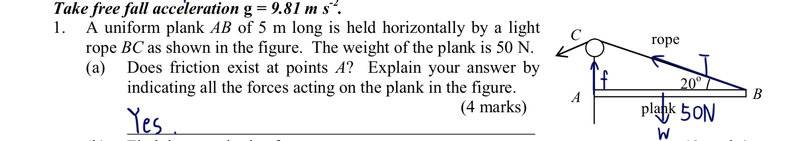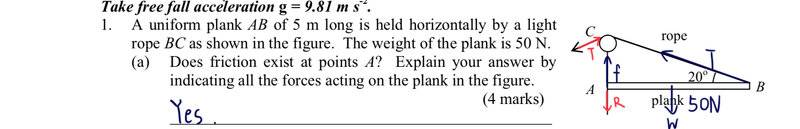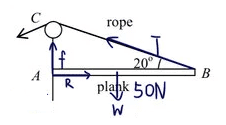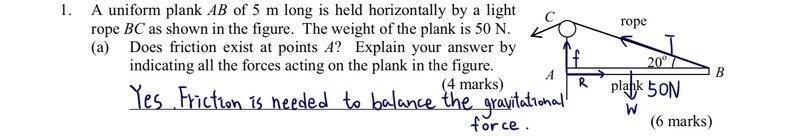# Question on Moment of a force

dorothy
Homework Statement:
I want to know whether I draw the free body diagram correctly? Thank you.

T = tension
f = friction
W = gravitational force
Relevant Equations:
/dorothy

Homework Helper
Gold Member
The forces that you have drawn look good. But you have left out a force. See if you can spot the missing force.

Also, you will want to explain from your diagram why there must be a friction force at point A.

dorothy
The forces that you have drawn look good. But you have left out a force. See if you can spot the missing force.

Also, you will want to explain from your diagram why there must be a friction force at point A.
Is it the normal reaction missing?

Homework Helper
Gold Member
Is it the normal reaction missing?
Yes. To be sure that you are thinking correctly, I would need to see where you place the force and the direction of the force.

dorothy
Yes. To be sure that you are thinking correctly, I would need to see where you place the force and the direction of the force.R stands for the normal reaction. Is it correct? also, I’ve added a new T for tension. Is the T in red needed？

Homework Helper
Gold Member
The reaction force R should be a normal force acting normal to the wall. I assume there is a wall.

The red tension force is not a force acting on the plank. So, it would not be included in a free-body diagram of the plank.

If this is a graded assignment, can you give us assurance that you are allowed to get external help? (I should have asked this at the beginning)

dorothy
The reaction force R should be a normal force.

The red tension force is not a force acting on the plank. So, it would not be included in a free-body diagram of the plank.

If this is a graded assignment, can you give us assurance that you are allowed to get external help? (I should have asked this at the beginning)
It’s not a graded assignment. It’s just a simple exercise because now I’m in vacation so I would like to do some more practice to train myself 😅

•TSny
dorothy
The reaction force R should be a normal force acting normal to the wall. I assume there is a wall.

The red tension force is not a force acting on the plank. So, it would not be included in a free-body diagram of the plank.

If this is a graded assignment, can you give us assurance that you are allowed to get external help? (I should have asked this at the beginning)

So I do draw the R correctly?

Homework Helper
Gold Member
So I do draw the R correctly?
No.
My interpretation of the diagram is that the left end of the plank is up against something like a wall. The wall exerts a force against the left end of the plank. This force from the wall has a component parallel to the wall (this is the friction force) and a component normal to the wall.

Homework Helper
Gold MemberLooks very good. Can you use this diagram to explain why the friction force cannot be zero and why it must be in the upward direction?

Last edited:
dorothy
Looks very good. Can you use this diagram to explain why the friction force cannot be zero and why it must be in the upward direction?
Is it because ‘friction has to be there to balance the gravitational force W ’?

Homework Helper
Gold Member
Is it because ‘friction has to be there to balance the reaction force in order to stay at rest’?
No. The friction force is vertical while the reaction force is horizontal. Since they are in different directions they cannot balance each other.

What are the basic conditions that the forces and the torques of the forces must obey in order for the plank to be in equilibrium?

dorothy
Is it because ‘friction has to be there to balance the gravitational force W ’?
I‘ve just change my thought , is it correct in this case?

dorothy
What are the basic conditions that the forces and the torques of the forces must obey in order for the plank to be in equilibrium?
The two forces should be parallel and with equal magnitude but opposite in direction

Homework Helper
Gold Member
The two forces should be parallel and with equal magnitude but opposite in direction
There are four forces acting on the plank. What condition or relation must be obeyed by these four forces if the plank is in equilibrium?

Is there also a condition involving torques?

Homework Helper
Gold Member
Take a look at your FBD and see whether or not each force has a counterpart which prevents the plank from moving in any direction, as it actually happens.
Remember, summation of forces along x-axis and y-axis must be zero, if balance exists.

If any of those forces is located at certain distance from a pivot point, it could make the body rotate about that point (not a balance condition); therefore, something must prevent that from happening.

dorothy
There are four forces acting on the plank. What condition or relation must be obeyed by these four forces if the plank is in equilibrium?

Is there also a condition involving torques?
Can I answer this question in this way?dorothy
There are four forces acting on the plank. What condition or relation must be obeyed by these four forces if the plank is in equilibrium?

Is there also a condition involving torques?
Net force=0?

dorothy
There are four forces acting on the plank. What condition or relation must be obeyed by these four forces if the plank is in equilibrium?

Is there also a condition involving torques?
Torque can be calculated by 5f?

Homework Helper
Gold Member
Can I answer this question in this way?View attachment 299700
No.
The tension force, T, has a vertical component. Perhaps this vertical component of T happens to cancel W. If so, the friction would not be needed. However, there is a way to show that f needed. Think about torques.

Homework Helper
Gold Member
Torque can be calculated by 5f?
I think we need to remember the basic conditions required for equilibrium:

(1) The vector sum of all of the forces acting on the plank must be zero.

(2) The sum of all of the torques acting on the plank must be zero for any choice of the location of the origin for calculating the torques.

Condition (1) can be restated as saying two things:
(1a) The sum of the horizontal components of the forces must add to zero.
(1b) The sum of the vertical components of the forces must add to zero.

We've already seen that (1b) doesn't give us enough information to decide whether or not the friction force is required. And (1a) won't be of any help since it deals with horizontal forces while f is vertical.

•Lnewqban
dorothy
I think we need to remember the basic conditions required for equilibrium:

(1) The vector sum of all of the forces acting on the plank must be zero.

(2) The sum of all of the torques acting on the plank must be zero for any choice of the location of the origin for calculating the torques.

Condition (1) can be restated as saying two things:
(1a) The sum of the horizontal components of the forces must add to zero.
(1b) The sum of the vertical components of the forces must add to zero.

We've already seen that (1b) doesn't give us enough information to decide whether or not the friction force is required. And (1a) won't be of any help since it deals with horizontal forces while f is vertical.
I get the concept but I don’t know how to explain it in Q1 🥲

Homework Helper
Gold Member
I get the concept but I don’t know how to explain it in Q1 🥲
Try picking point B as an origin for the torques.

(1) Does the tension force T produce any torque about B? If so, is the torque clockwise or counterclockwise?

(2) Does the weight W produce any torque about B? If so, is the torque clockwise or counterclockwise?

(3) Does the reaction force R produce any torque about B? If so, is the torque clockwise or counterclockwise?

Based on your answers to these three questions, can you argue that there must be a friction force at A and that the friction force must be upward?

dorothy
Try picking point B as an origin for the torques.

(1) Does the tension force T produce any torque about B? If so, is the torque clockwise or counterclockwise?

(2) Does the weight W produce any torque about B? If so, is the torque clockwise or counterclockwise?

(3) Does the reaction force R produce any torque about B? If so, is the torque clockwise or counterclockwise?

Based on your answers to these three questions, can you argue that there must be a friction force at A and that the friction force must be upward?
1. Yes, clockwise
2. Yes, anti-clockwise
3. No
Ohhh is it because we need the moment due to f to balance with the moment due to W in order to reach the equilibrium?

Homework Helper
Gold Member
1. Yes, clockwise
2. Yes, anti-clockwise
3. No
Ohhh is it because we need the moment due to f to balance with the moment due to W in order to reach the equilibrium?
You have the right ideaBut, the tension force T does not produce any torque about the origin at B. Can you see why?
This is important in order to be able to logically deduce that there must be an upward friction force.

dorothy
You have the right ideaBut, the tension force T does not produce any torque about the origin at B. Can you see why?
This is important in order to be able to logically deduce that there must be an upward friction force.
because the force T is pointing upward and it doesn’t “push” the plank?

Homework Helper
Gold Member
because the force T is pointing upward and it doesn’t “push” the plank?
No.

Have you covered the concept of "line of action of a force"? What can you say about the torque of a force about some origin if the line of action of the force passes through the origin?

I'm curious as to your reason for stating (correctly!) that the torque due to R about point B is zero.

dorothy
No.

Have you covered the concept of "line of action of a force"? What can you say about the torque of a force about some origin if the line of action of the force passes through the origin?

I'm curious as to your reason for stating (correctly!) that the torque due to R about point B is zero.
oh because I think that T is pointing upward so it won’t pass B. But for R, it is pointing horizontally and will pass B so its torque is 0.

Homework Helper
Gold Member
oh because I think that T is pointing upward so it won’t pass B. But for R, it is pointing horizontally and will pass B so its torque is 0.
Good reasoning for R, but not for T. If you drew a line through T, the line would pass through B. So, just like R, the line of action passes through B. So, the torque about B due to T is zero.

It should be clear that: any force that acts at the origin would not produce any torque about that origin since the line of action of the force clearly passes through the origin.

•